Courses

# Field Balancing Mechanical Engineering Notes | EduRev

## Mechanical Engineering : Field Balancing Mechanical Engineering Notes | EduRev

The document Field Balancing Mechanical Engineering Notes | EduRev is a part of Mechanical Engineering category.
All you need of Mechanical Engineering at this link: Mechanical Engineering

Field Balancing of a Rotor

• Method of balancing a rotor is based on an extension of balancing of a thin disc during rotation
• Following the text book "Principles of Vibration Control", one can show that for balancing of thin disc one needs to measure
• a. amplitude (Ai ) and phase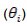of vibration
b. the effect of adding additional mass ( Mt) to the disc for the amplitude (At) and phase (φt).
• Accordingly, denoting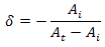one can find the balancing mass ( mb) and it's location (rb, φb) in polar coordinates as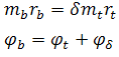• For balancing of a rotor one needs three trest runs and measure the amplitude and phase of vibration at two different locations 1 and 2.

• At first, amplitudes and phases corresponding to unbalanced rotor at locations 1 and 2 denoted as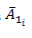and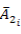are measured.

• Next for a trial mass the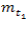same are noted. Let these be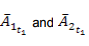• Finally, in the third run, for a trial mass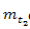one needs to obtain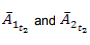• One can show that the balancing masses required are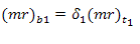and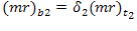where,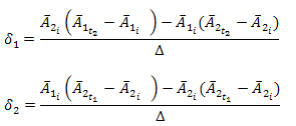where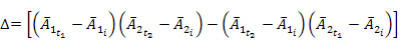A rotor operating at a speed higher than (or close to) its first critical speed is termed as a flexible rotor since it undergoes a significant transverse deflection at this speed.

• Unlike a rigid rotor, a flexible rotor cannot be balanced by adding two masses placed in two arbitrarily chosen planes.

• The principle used for balancing a flexible rotor is entirely different from that applied in the case of a rigid rotor. The objective of attaching the balancing masses to a rigid rotor, as already stated, is to neutralize the unbalanced forces and moments.

• In a flexible rotor, on the other hand, the balancing masses are attached to suitably modify the dynamic deflection characteristics of the rotor. The technique to do this is known as the modal balancing technique .
Offer running on EduRev: Apply code STAYHOME200 to get INR 200 off on our premium plan EduRev Infinity!

,

,

,

,

,

,

,

,

,

,

,

,

,

,

,

,

,

,

,

,

,

;